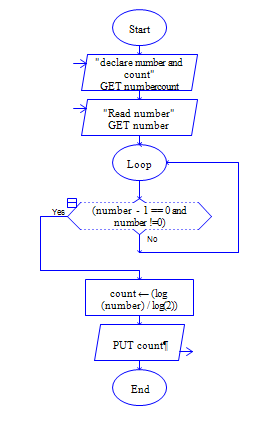# Program to check whether given Integer is whether integer is power of 2 or not

Sample Input 1 :

64

Sample Output 1 :

64 is Power of 2 (2*2*2*2*2*2=64)

#### Flow Chart Design#### Program or Solution

``` #include<stdio.h> int main() { int number, n,i,flag=0; printf("Enter a Number: "); scanf("%d",&number); n=number; while(n>1) { if(n%2!=0) { flag=1; break; } n=n/2; } if(flag==0) { printf("%d is a power of 2 ",number); } else { printf("%d is not power of 2 ",number); } return 0; } ```

#### Program Explanation

refer video tutorial